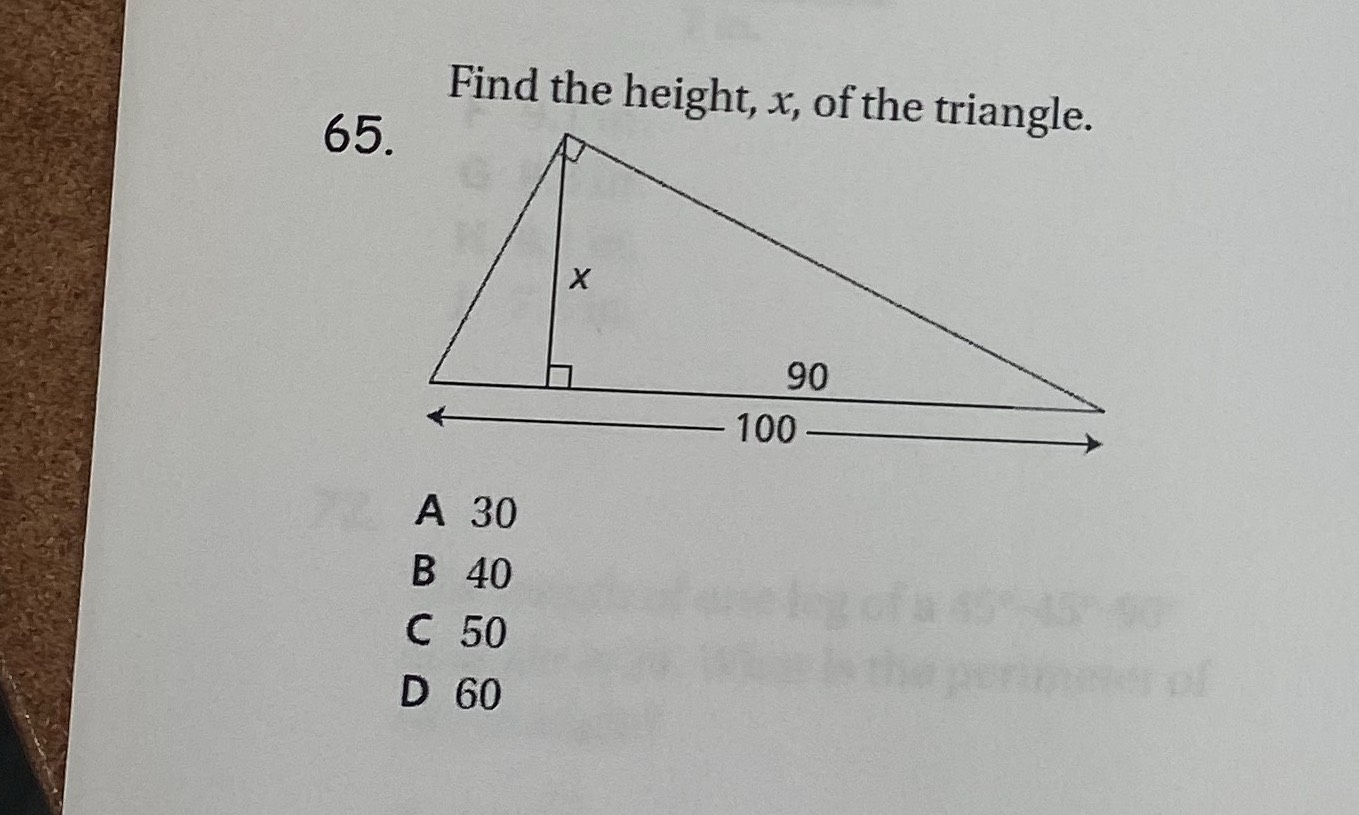### Still have math questions?

Geometry
QuestionFind the height, $$x$$, of the triangle.

$$30$$

$$40$$

$$50$$

$$60$$

$$30$$